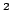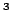Statistica Sinica 18(2008), 515-534

REGRESSION FOR LONGITUDINAL/CLUSTERED DATA

Kani Chen, Jianqing Fanand Zhezhen JinHong Kong University of Science and Technology,Princeton University

andColumbia University

Abstract: This paper studies a weighted local linear regression smoother for longitudinal/clustered data, which takes a form similar to the classical weighted least squares estimate. As a hybrid of the methods of Chen and Jin (2005) and Wang (2003), the proposed local linear smoother maintains the advantages of both methods in computational and theoretical simplicity, variance minimization and bias reduction. Moreover, the proposed smoother is optimal in the sense that it attains linear minimax efficiency when the within-cluster correlation is correctly specified. In the special case that the joint density of covariates in a cluster exists and is continuous, any working within-cluster correlation would lead to linear minimax efficiency for the proposed method.

Key words and phrases: Asymptotic bias, generalized estimating equations, kernel function, linear minimax efficiency, mean squared error, nonparametric curve estimation.